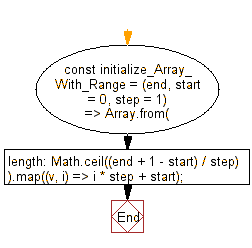# JavaScript: Initialize an array containing the numbers in the specified range where start and end are inclusive with their common difference step

## JavaScript fundamental (ES6 Syntax): Exercise-54 with Solution

Write a JavaScript program to initialize an array containing the numbers in the specified range where start and end are inclusive with their common difference step.

• Use Array.from() to create an array of the desired length.
• Use (end - start + 1)/step and a map function to fill the array with the desired values in the given range.
• Omit the second argument, start, to use a default value of 0.
• Omit the last argument, step, to use a default value of 1.

Sample Solution:

JavaScript Code:

``````//#Source https://bit.ly/2neWfJ2
const initialize_Array_With_Range = (end, start = 0, step = 1) =>
Array.from({ length: Math.ceil((end + 1 - start) / step) }).map((v, i) => i * step + start);

console.log(initialize_Array_With_Range(5));
console.log(initialize_Array_With_Range(8, 3));
console.log(initialize_Array_With_Range(6, 0, 2));
```
```

Sample Output:

```[0,1,2,3,4,5]
[3,4,5,6,7,8]
[0,2,4,6]
```

Pictorial Presentation:Flowchart:Live Demo:

See the Pen javascript-basic-exercise-1-54 by w3resource (@w3resource) on CodePen.

Improve this sample solution and post your code through Disqus

What is the difficulty level of this exercise?

Test your Programming skills with w3resource's quiz.

﻿

## JavaScript: Tips of the Day

Chunks an array into n smaller arrays

Example:

```const tips_chunkIntoN = (arr, n) => {
const size = Math.ceil(arr.length / n);
return Array.from({ length: n }, (v, i) =>
arr.slice(i * size, i * size + size)
);
}
console.log(tips_chunkIntoN([1, 2, 3, 4, 5, 6, 7,8], 4));
```

Output:

```[[1,2],[3,4],[5,6],[7,8]]
```